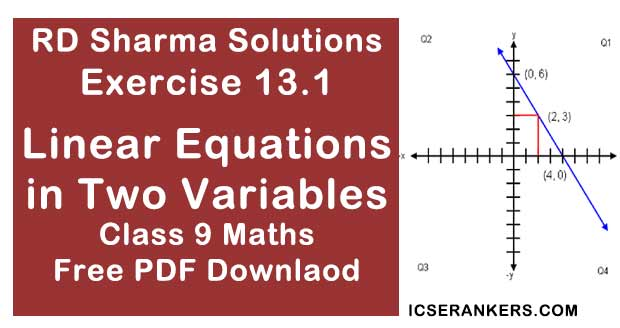# Chapter 13 Linear Equations in Two Variables RD Sharma Solutions Exercise 13.1 Class 9 MathsChapter Name RD Sharma Chapter 13 Linear Equations in Two Variables Exercise 13.1 Book Name RD Sharma Mathematics for Class 10 Other Exercises Exercise 13.2Exercise 13.3Exercise 13.4 Related Study NCERT Solutions for Class 10 Maths

### Exercise 13.1 Solutions

1. Express the following linear equations in the form ax + by + c = 0 and indicate the values of a, b and c in each case :

(i) -2x + 3y = 12
(ii) x - y/2 - 5 = 0
(iii) 2x + 3y = 9.35
(iv) 3x = -7y

Solution

(i)  We have
-2x + 3y = 12
⇒ -2x + 3y - 12 = 0
On comparing this equation with ax + by + c = 0  we obtain a = -2, b = 3 and c = -12.

(ii) Given that
x - y/2 - 5 = 0
1x - y/2 - 5 = 0
On comparing this equation with ax + by + c = 0 we obtain a = 1, b = -1/2 and c = -5

(iii) Given that
2x + 3y = 9.35
⇒ 2x + 3y - 9.35 = 0
On comparing this equation with ax + by +c = 0 we get a  = 2, b = 3 and c = 9.35

(iv) 3x = -7y ⇒ 3x + 7y + 0 = 0
On comparing this equation with ax + by + c = 0 we get a = 3, b = 0 and c = 3

(v) We have
2x + 3 = 0
2x + 0(y) + 3 = 0
On comparing this equation with ax + by + c = 0 we get a = 2, b = 0 and c = 3

(vi) Given that
y - 5 = 0
⇒ 0x + 1y - 5 = 0
On comparing this equation with ax + by + c = 0 we get a = 0, b = 1 and c = -5

(vii) We have
4 = x
-3x + 0.y + 4 = 0
On comparing the equation with ax + by + c = 0 we get a = -3, b = 0 and c = 4

(viii) Given that,
y = x/2
⇒ 2y = x
⇒ x - 2y + 0 = 0
On comparing this equation with ax + by + c = 0 we get a = 1, b = -2 and c = 0

2. Write each of the following as an equation in two variables:
(i) 2x = - 3
(ii) y = 3
(iii) 5x = 7/2
(iv) y = 3x/2

Solution

(i) We have
2x = - 3
⇒ 2x + 3 = 0
⇒ 2x + 0.y + 3 = 0

(ii) We have,
y = 3
y - 3 = 0
⇒ 0.x + 1.y - 3 = 0

(iii) Given
5x = 7/2
10x - 7 = 0
10x + 0.y - 7 = 0

(iv) We have
y = 3x/2
3x - 2y = 0
3x - 2y + 0 = 0

3. The cost of ball pen is Rs. 5 less than half of the cost of fountain pen. Write this statement as a linear equation in two variables.

Solution

Let us assume the cost of the ball pen be Rs. x and that of a fountain pen to be y then according to given statements
We have
x = y/2 - 5
⇒ 2x = y - 10
⇒ 2x  - y + 10 = 0